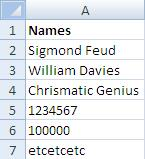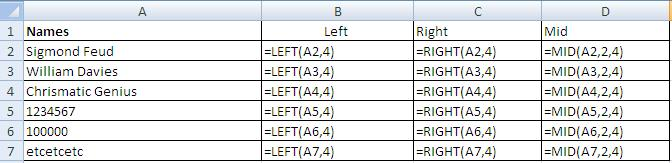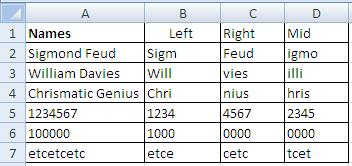# MS Excel left right mid function to extract text

## How to extract certain Text from Cells of MS excel to extract characters from right, left or centre of the data in a particular cell of MS excel.

MS Excel left right mid function to extract text: MS Excel left right mid function to extract text: This is really easy to get or extract specific text or characters from MS excel cell. Illustration is given below. Illustration for MS Excel Extract Text using Left, Right, or mid function:

Let us assume we need to obtain only the first 4 characters of the given cells from below table:

### MS Excel left right mid function to extract textWe shall use “left” function if we desire to obtain first four characters from left side. We may also use “MS excel right” function if we wish to get first four characters from the right side. We also have the option to use “MS Excel mid” function to extract desired number of characters from the centre of any cell. Let us now begin with by obtaining all the three in below table:MS Excel left right mid function to extract text-2

### Formula for MS Excel Left Right and mid function:MS Excel left right mid function to extract text-3

MS excel left and right functions are pretty simple because they require only the selection of cell and number of characters to be extracted but MS Mid function is different because it requires three things:

1) Cell from where data is to be extracted
2) Number of the character(from left) from where to begin extracting data
3) Number of characters after which it is to stop extraction.

In above example, =MID(A2, defines only the selection of cell from where data is to be retrieved while =MID(A2,2 after comma is meant for defining the starting number of character to begin extraction i.e. in A2 the MID function will begin to extract characters from 2nd character “i” up to “o”.
Final result of the data extraction is placed below: